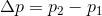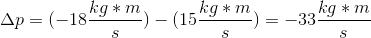AP Physics B : Understanding Conservation of Momentum

Example Questions

Example Question #1 : Momentum

A baseball pitcher throws a baseball at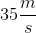horizontally in the positive direction to a batter, who hits the ball in the opposite direction at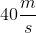. What is the change in momentum of the baseball if the baseball has a mass of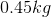?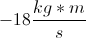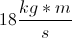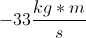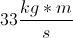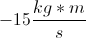Explanation:

In order to calculate the change in momentum, we must find the initial and final momentum of the baseball, and then find the difference.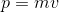Use the given velocities and mass to calculate the initial and final values.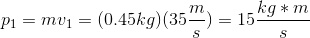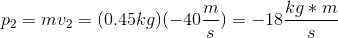The initial momentum is positive because the problem states that the ball was originally thrown in the positive direction. The final momentum is negative due to the change in direction.

Now we find the change in momentum: